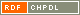# Show document

Title: Permanent versus determinant over a finite field Dolinar, Gregor (Author)Guterman, Aleksandr Èmilevič (Author)Orel, Marko (Author)Kuzma, Bojan (Author)http://dx.doi.org/10.1007/s10958-013-1469-4 English Not categorized 1.08 - Published Scientific Conference Contribution IAM - Andrej Marušič Institute Let ▫$\mathbb{F}$▫ be a finite field of characteristic different from 2. We study the cardinality of sets of matrices with a given determinant or a given permanent for the set of Hermitian matrices ▫$\mathcal{H}_n(\mathbb{F})$▫ and for the whole matrix space ▫$M_n(\mathbb{F})$▫. It is known that for ▫$n = 2$▫, there are bijective linear maps ▫$\Phi$▫ on ▫$\mathcal{H}_n(\mathbb{F})$▫ and ▫$M_n(\mathbb{F})$▫ satisfying the condition per ▫$A = \det \Phi(A)$▫. As an application of the obtained results, we show that if ▫$n \ge 3$▫, then the situation is completely different and already for ▫$n = 3$▫, there is no pair ofmaps ▫$(\Phi, \phi)$▫, where ▫$\Phi$▫ is an arbitrary bijective map on matrices and ▫$\phi \colon \mathbb{F} \to \mathbb{F}$▫ is an arbitrary map such that per ▫$A = \phi(\det \Phi(A))$▫ for all matrices ▫$A$▫ from the spaces ▫$\mathcal{H}_n(\mathbb{F})$▫ and ▫$M_n(\mathbb{F})$▫, respectively. Moreover, for the space ▫$M_n(\mathbb{F})$▫, we show that such a pair of transformations does not exist also for an arbitrary ▫$n > 3$▫ if the field ▫$\mathbb{F}$▫ contains sufficiently many elements (depending on ▫$n$▫). Our results are illustrated by a number of examples. mathematics, linear algebra, matrix theory, permanent, determinant 2013 Str. 404-413 1072-3374 512.643 16715865810 66Document is not linked to any category.

Average score: (0 votes) Voting is allowed only to logged in users.Hover the mouse pointer over a document title to show the abstract or click on the title to get all document metadata.

## Secondary language

Language: Slovenian Permanente v primerjavi z detminantami nad končnimi polji Naj bo ▫$\mathbb{F}$▫ končno polje, katerega karakteristika ni enaka 2. V članku obravnavamo kardinalnost množice matrik, ki imajo enako predpisano vrednost determinante, in kardinalnost množice matrik, ki imajo enako predpisano vrednost permanente. Pri tem se najprej omejimo na hermitske matrike ▫$\mathcal{H}_n(\mathbb{F})$▫, nato pa obravnavamo problem še v okviru množice vseh matrik ▫$M_n(\mathbb{F})$▫. Znano je, da za ▫$n = 2$▫ obstajajo take bijektivne linearne preslikave ▫$\Phi$▫ na ▫$\mathcal{H}_n(\mathbb{F})$▫ in ▫$M_n(\mathbb{F})$▫, da velja ▫$\text{per} A = \det \Phi(A)$▫. S pomočjo dobljenih rezultatov pokažemo, da je za ▫$n \ge 3$▫ situacija povsem drugačna. Že za ▫$n = 3$▫ ne obstaja noben par preslikav ▫$(\Phi, \phi)$▫, kjer bi bila ▫$\Phi$▫ poljubna bijektivna preslikava na matrikah in bi bila ▫$\phi \colon \mathbb{F} \to \mathbb{F}$▫ poljubna preslikava, tako da bi veljalo ▫$\text{per} A = \phi(\det \Phi(A))$▫ za vse matrike ▫$A$▫ iz prostora ▫$\mathcal{H}_n(\mathbb{F})$▫ ali ▫$M_n(\mathbb{F})$▫. Še več, za prostor ▫$M_n(\mathbb{F})$▫ pokažemo, da tak par preslikav ne obstaja za poljuben ▫$n > 3$▫, če polje ▫$\mathbb{F}$▫ vsebuje dovolj elementov (v odvisnosti od ▫$n$▫). Navedenih je tudi več primerov, ki ilustrirajo naše rezultate. matematika, linearna algebra, teorija matrik, permanenta, determinanta